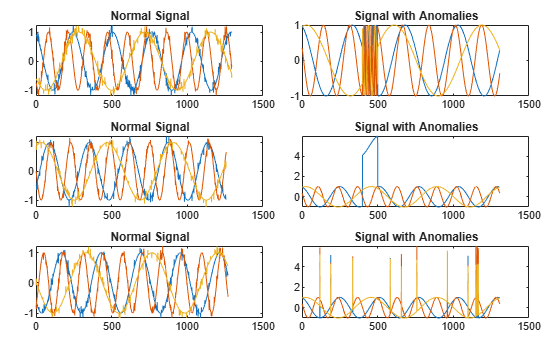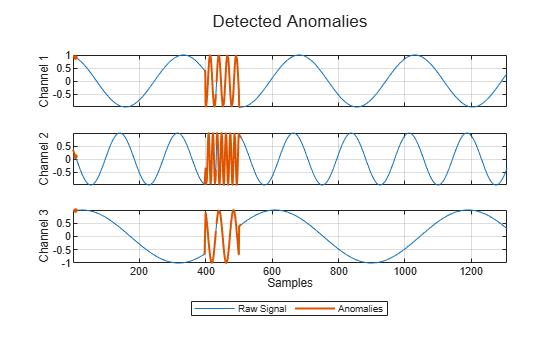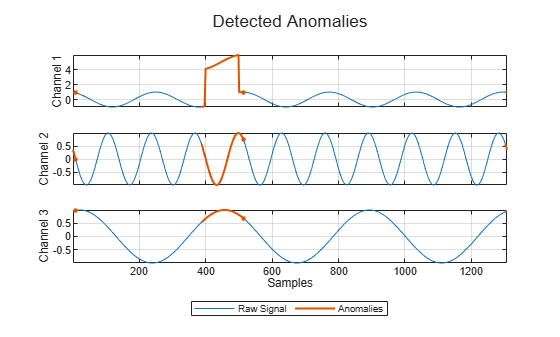# plotAnomalies

Plot signal anomalies

Since R2023a

## Syntax

``plotAnomalies(d,s)``
``plotAnomalies(d,s,Name=Value)``
``f = plotAnomalies(___)``

## Description

example

````plotAnomalies(d,s)` plots the anomalies in signal observation `s` detected by the detector `d`. NoteTo plot signal anomalies, the detector must be trained. ```
````plotAnomalies(d,s,Name=Value)` specifies additional options using name-value arguments.```
````f = plotAnomalies(___)` returns the figure handle of the plot.```

## Examples

collapse all

Load a convolutional anomaly detector trained with three-channel sinusoidal signals. Display the model, threshold, and window properties of the detector.

```load sineWaveAnomalyDetector D```
```D = deepSignalAnomalyDetectorCNN with properties: IsTrained: 1 NumChannels: 3 Model Information ModelType: 'conv' FilterSize: 8 NumFilters: 32 NumDownsampleLayers: 2 DownsampleFactor: 2 DropoutProbability: 0.2000 Threshold Information Threshold: 0.0510 ThresholdMethod: 'contaminationFraction' ThresholdParameter: 0.0100 Window Information WindowLength: 1 OverlapLength: 'auto' WindowLossAggregation: 'mean' ```

Load the file `sineWaveAnomalyData.mat`, which contains two sets of synthetic three-channel sinusoidal signals.

• `sineWaveNormal` contains the 10 sinusoids used to train the convolutional anomaly detector. Each signal has a series of small-amplitude impact-like imperfections but otherwise has stable amplitude and frequency.

• `sineWaveAbnormal` contains three signals of similar length and amplitude to the training data. One of the signals has an abrupt, finite-time change in frequency. Another signal has a finite-duration amplitude change in one of its channels. A third has random spikes in each channel.

Plot three normal signals and the three signals with anomalies.

```load sineWaveAnomalyData tiledlayout(3,2,TileSpacing="compact",Padding="compact") rnd = randperm(length(sineWaveNormal)); for kj = 1:length(sineWaveAbnormal) nexttile plot(sineWaveNormal{rnd(kj)}) title("Normal Signal") nexttile plot(sineWaveAbnormal{kj}) title("Signal with Anomalies") end```Use the trained anomaly detector to detect the anomalies in the abnormal data. Use the `plotAnomalies` function to plot each channel of each signal and annotate the anomalies found by the detector. The anomalies are assigned to all channels of a signal even when they are present just in one channel.

```for kj = 1:length(sineWaveAbnormal) figure plotAnomalies(D,sineWaveAbnormal{kj}) end```## Input Arguments

collapse all

Anomaly detector, specified as a `deepSignalAnomalyDetectorCNN` or `deepSignalAnomalyDetectorLSTM` object. Use the `deepSignalAnomalyDetector` function to create `d`.

Signal observation, specified as a vector.

### Name-Value Arguments

Specify optional pairs of arguments as `Name1=Value1,...,NameN=ValueN`, where `Name` is the argument name and `Value` is the corresponding value. Name-value arguments must appear after other arguments, but the order of the pairs does not matter.

Example: `PlotReconstruction=true,ExecutionEnvironment="cpu"` instructs the function to plot the reconstructed signal and use the computer CPU to detect anomalies.

Option to plot the reconstructed signal, specified as either `false` or `true`.

Data Types: `logical`

Label priority for overlapped windows, specified as `"anomaly"` or `"normal"`.

• If you specify `OverlapPriority` as `"anomaly"`, the function labels samples in an abnormal window overlapped with a normal window as abnormal.

• If you specify `OverlapPriority` as `"normal"`, the function labels samples in a normal window overlapped with an abnormal window as normal.

This argument applies only the window overlap length is greater than zero.

Data Types: `char` | `string`

Execution environment used by the network, specified as one of these:

• `"auto"` — If available, use the GPU. If the GPU is not available, use the CPU.

• `"gpu"` — Use the GPU.

• `"cpu"` — Use the CPU.

Data Types: `char` | `string`

## Output Arguments

collapse all

Figure handle of the plot, returned as a figure handle.

## Version History

Introduced in R2023a